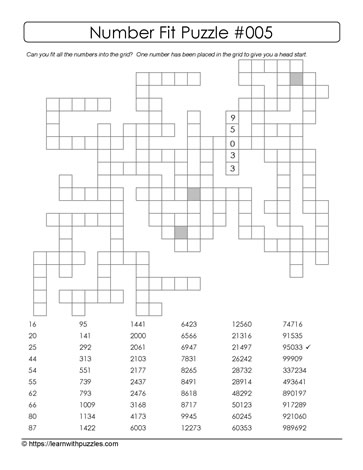## Number Fit Puzzle - 005

()Number Fit Puzzle - 005 For Google Apps

Here is a number fit puzzle, similar to the freeform crossword puzzles, only this puzzle form uses numbers that range from 2-digit to 6-digits.

Use the 5-digit number hint to start solving the puzzle.

This puzzle supports numeration and number sense strand in math for students learning numbers to 1,000,000. And, also provides extension activities for students requiring differentiated instruction.

For example: students who have learned numbers to 100,000 might find this puzzle a worthwhile challenge to see if they can:
l. write the number words for the 6-digit numbers;
2. orally present the list of 6-digit numbers;
3. use the 4 different operations and create math questions to support and consolidate learning